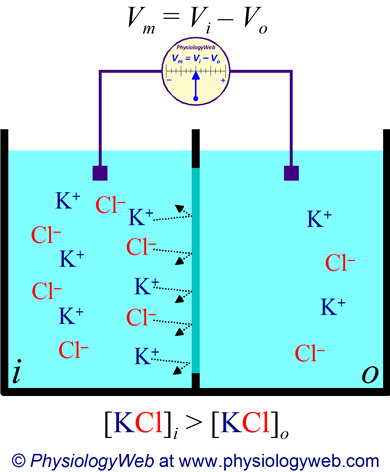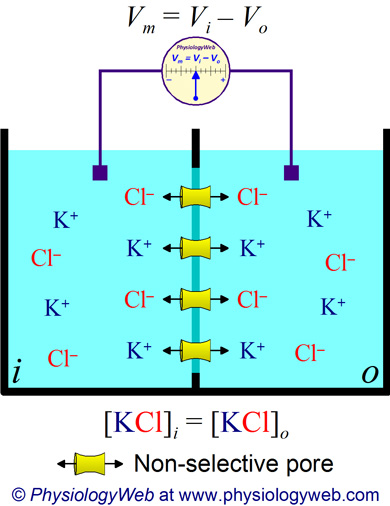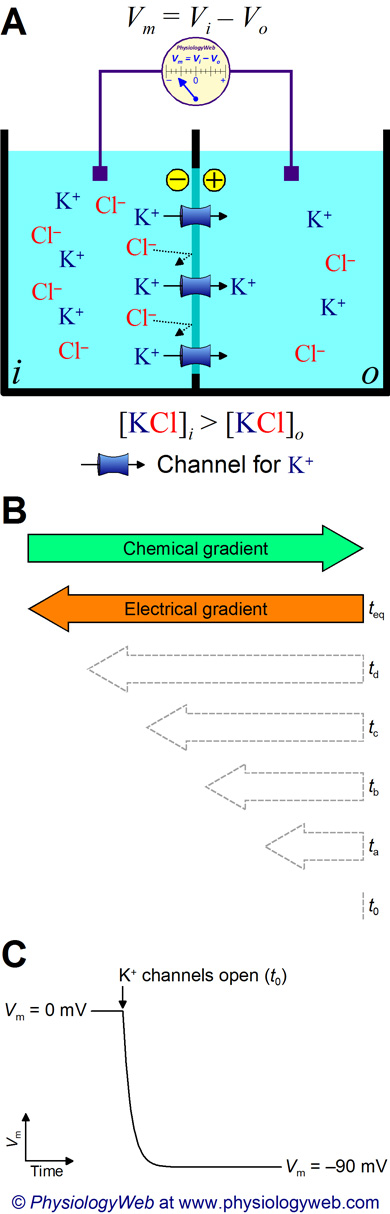PhysiologyWeb  Home  |  FAQ  |  About  |  Contact  |   - Sign InTweetResting Membrane Potential -
Establishment of the Membrane Potential
Our goal is to first understand how the membrane potential comes about. Later, we will discuss the factors that govern the value of the membrane potential. Two factors are important for the generation of the membrane potential. In fact, these two factors are the minimum essential features that must be present in a system before a membrane potential can be established. The two factors are:
1. Asymmetric distribution of ions across the plasma membrane (i.e., ion concentration gradients); and
2. Selective ion channels in the plasma membrane. K+, Na+, and Cl channels are the most important channel types for most cells; however, there are many cells in which other channels are important as well.
Lets see how the membrane potential can be established in the first place. Later, we will see how we can calculate and even manipulate the value of the membrane potential. We will start by considering a hypothetical example of two solution compartments separated by a membrane. This example can later be extended to understand how the membrane potential is established in real cells. Assume that we have two solution compartments that are separated by a membrane, whose lipid and protein compositions can be altered depending on the unique cases considered (see Figs. 1–3). Because we want to understand the situation in biological cells, we will designate one compartment as the inside or intracellular compartment (i in Figs. 1–3) and another as the outside or extracellular compartment (o in Figs. 1–3). These compartments represent the intracellular and extracellular fluid compartments, respectively. In the intracellular compartment (i), we place a solution of 150 mM KCl. In the extracellular compartment (o), we have 5 mM KCl. Finally, we will set up a simple apparatus to measure the potential difference (membrane potential of Vm) across the membrane that separates the two compartments.
Here, our focus will be on K+ ions, and we have chosen these concentrations to resemble the physiological concentrations of K+ in intracellular and extracellular solutions. Also for the sake of this exercise, we will ignore osmotic forces that are caused by the differences in the concentration of solutes across the membrane. In real cells, the osmolarity of the intracellular and extracellular solutions must, of course, be the same in order to avoid cell swelling or shrinkage. Also, please remember that in real cells, the majority of intracellular anions are in fact carried on large proteins and, moreover, the extracellular Cl concentration is much higher (~110 mM). However, the KCl solutions used here have been chosen to simplify the numbers and, as we will see later in this lecture, the argument used here applies directly to real cells.Figure 1. Two solution compartments separated by a pure lipid bilayer.
If two solution compartments are separated by a pure lipid bilayer, there can be no movement of ions across this bilayer from one compartment to another, even if a concentration gradient exists between the compartments. Therefore, no separation of charge can take place across this pure lipid bilayer and, consequently, no voltage difference (i.e., membrane potential) can be established across this membrane. Thus, Vm = 0 mV in this scenario.
Let's first consider the situation where the membrane separating the two compartments is a pure lipid bilayer (i.e., devoid of membrane proteins) (Fig. 1). In this case, the voltage difference between the two compartments (i.e., the membrane potential) is zero. This is because in each compartment electroneutrality is perfectly obeyed. That is in each compartment, the sum of positive charges equals the sum of negative charges. Although, the KCl concentration is higher in the intracellular compartments, no diffusion of ions can occur due to the fact that no ionic pathways are present in the membrane for K+ and Cl. Remember that ions cannot cross biological membranes without the aid of membrane transport proteins. Therefore, there can be no charge separation across the membrane and, therefore, there is no potential difference between the two compartments (Fig. 1). Although there is a concentration gradient, because there are no ionic pathways to allow the passage of ions, charge separation across the membrane cannot be established. Remember that a potential difference can only be established if there is charge separation across the membrane.
We now introduce large holes in the lipid bilayer that separates the two compartments (Fig. 2). Assume that the holes are large in diameter and non-selective, which will allow all solutes to pass through. In this case, both K+ and Cl diffuse from compartment i to o down their respective concentration gradients. In order to maintain electroneutrality, the same number of K+ and Cl move together from i to o. The movement of K+ and Cl from i to o will continue until equilibrium is established. At equilibrium, the KCl concentration will be the same in both compartments, and will be the algebraic average of the initial values ([KCl]i = [KCl]o = 77.5 mM). Again, electroneutrality is maintained in both compartments. No charge separation has taken place across the lipid bilayer and, thus, no voltage difference exists across the membrane. It is emphasized again that a potential difference can only be established if there is charge separation across the membrane.Figure 2. Two solution compartments separated by a membrane that contains non-selective pores.
If two solution compartments are separated by a membrane that contains non-selective pores, each solute diffuses down its own concentration gradient until the solute concentration is the same on both sides (i.e., until the concentration gradient is dissipated). Because of the non-selective nature of the pores, movement of K+ from i to o will be followed and balanced by Cl. Therefore, no separation of charge can take place across this membrane and, consequently, no voltage difference (i.e., membrane potential) can be established across this membrane. Thus, Vm = 0 mV in this scenario.
It is important to note that prior to the opening of the K+ channels, no potential difference existed between the two compartments (i.e., Vm = 0 mV). As soon as the first K+ ion diffuses from i to o, a small membrane potential is established, which grows in size until the magnitude of the electrical gradient equals the magnitude of the chemical gradient (Fig. 3, B and C). When the electrical gradient exactly balances the chemical gradient, K+ is said to be at electrochemical equilibrium. Based on the intracelluar and extracellular concentrations of K+ used in this example, the final (i.e., equilibrium) value of the membrane potential is about −90 mV (intracellular side negative with respect to the extracellular side). In the next two sections of this lecture, we will learn how to calculate the value of the membrane potential.
To summarize, while K+ is diffusing from i to o down its concentration gradient, a negative membrane potential is being established that favors the retention of K+ in compartment i. Therefore, two forces are now acting on K+:Figure 3. Two solution compartments separated by a membrane that contains potassium (K+) channels.